## 4.2.2 3D Rigid Bodies: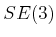One might expect that definingfor a 3D rigid body is an obvious extension of the 2D case; however, 3D rotations are significantly more complicated. The resulting C-space will be a six-dimensional manifold,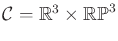. Three dimensions come from translation and three more come from rotation.

The main quest in this section is to determine the topology of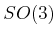. In Section 3.2.3, yaw, pitch, and roll were used to generate rotation matrices. These angles are convenient for visualization, performing transformations in software, and also for deriving the DH parameters. However, these were concerned with applying a single rotation, whereas the current problem is to characterize the set of all rotations. It is possible to use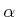,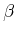, and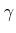to parameterize the set of rotations, but it causes serious troubles. There are some cases in which nonzero angles yield the identity rotation matrix, which is equivalent to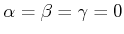. There are also cases in which a continuum of values for yaw, pitch, and roll angles yield the same rotation matrix. These problems destroy the topology, which causes both theoretical and practical difficulties in motion planning.

Consider applying the matrix group concepts from Section 4.2.1. The general linear group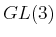is homeomorphic to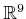. The orthogonal group,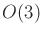, is determined by imposing the constraint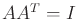. There are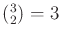independent equations that require distinct columns to be orthogonal, and three independent equations that force the magnitude of each column to be. This means thathas three dimensions, which matches our intuition since there were three rotation parameters in Section 3.2.3. To obtain, the last constraint,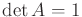, is added. Recall from Example 4.12 that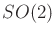consists of two circles, and the constraintselects one of them. In the case of, there are two three-spheres,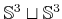, andselects one of them. However, there is one additional complication: Antipodal points on these spheres generate the same rotation matrix. This will be seen shortly when quaternions are used to parameterize.

Subsections
Steven M LaValle 2020-08-14